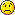- DSP log - http://www.dsplog.com -

Trying out PAPR reduction for OFDM by multiplication with j

Posted By Krishna Sankar On July 2, 2008 @ 6:08 am In OFDM | 47 Comments

In this post, we will explore a probable way of reducing PAPR (peak to average power ratio) in OFDM by changing the phase of some of the subcarriers. This is in response to the comment to post on Peak to Average power ratio for OFDM , where Mr. Elibom suggested to reduce the PAPR by cyclically rotate some of the subcarriers and using.

Further, the presentation in the IEEE TGN, PAPR in HT-LTF (11-06/1595r1) , mentions that in 40MHz mode where a 128pt FFT is used, PAPR of HT-LTF (High Throughput Long Training Field) can be reduced by multiplying the upper 20MHz subcarriers by j. Using quick Matlab simulations, we will try to validate that claim for HT-LTF and further check the PAPR for a general random BPSK and QPSK modulation.

## PAPR in HT-LTF

Joint Proposal PHY specification document (11-05/1102r4) . Note: The specification has evolved considerably over the past 2.5 years (and is not yet publically available), but for discussion on HT-LTF this document should suffice.

The peak to average power ratio for a signal is defined as
, where
corresponds to the conjugate operator.

Expressing in deciBels,
.

```Matlab/Octave script for computing PAPR in HT-LTF close all; clear all; nFFT = 128; nDSC = 114; % The 128pt HT-LTF sequence for 40MHz as defined in 11-06/1595r4 htLTF = [ zeros(1,6) 1 1 -1 -1 1 1 -1 1 -1 1 1 1 1 1 1 -1 -1 1 1 -1 1 -1 1 1 1 1 1 ... 1 -1 -1 1 1 -1 1 -1 1 -1 -1 -1 -1 -1 1 1 -1 -1 1 -1 1 -1 1 1 1 1 -1 -1 -1 1 0 ... 0 0 -1 1 1 -1 1 1 -1 -1 1 1 -1 1 -1 1 1 1 1 1 1 -1 -1 1 1 -1 1 -1 1 1 1 1 1 ... 1 -1 -1 1 1 -1 1 -1 1 -1 -1 -1 -1 -1 1 1 -1 -1 1 -1 1 -1 1 1 1 1 zeros(1,5)]; ```

``` x1F = htLTF; % no rotation x2F = [htLTF(1:64) j*htLTF(65:128)]; % with 90degree rotation ```

``` % Taking iFFT, time domain x1t = (nFFT/sqrt(nDSC))*ifft(fftshift(x1F.')).'; % no rotation x2t = (nFFT/sqrt(nDSC))*ifft(fftshift(x2F.')).'; % with rotation ```

``` % computing the peak to average power ratio for symbol without rotation meanSquareValue1 = sum(x1t.*conj(x1t),2)/nFFT; peakValue1 = max(x1t.*conj(x1t),[],2); paprSymbol1 = peakValue1./meanSquareValue1; paprSymbol1dB = 10*log10(paprSymbol1) % computing the peak to average power ratio for symbol with 90 degree rotation meanSquareValue2 = sum(x2t.*conj(x2t),2)/nFFT; peakValue2 = max(x2t.*conj(x2t),[],2); paprSymbol2 = peakValue2./meanSquareValue2; paprSymbol2dB = 10*log10(paprSymbol2)```

As claimed in slide7 of power point presentation PAPR In HT-LTF (11-06/1595r1) , the PAPR with

(a) No rotation is 5.6317dB and

(b) With 90 degree rotation is 3.4066dB.

So, around 2.2dB reduction in PAPR is achieved.## PAPR for random BPSK and QPSK

Armed with the above result, I tried to simulate the PAPR for with random BPSK (and QPSK) modulation on a 128pt FFT with and without rotating the upper 20MHz subcarriers by j.

The results from the simulations is shown below.

Figure: OFDM PAPR for BPSK/QPSK with j rotation

Observations

1. For random BPSK sequence, the multiplication by j resulted in increase of PAPR by around 0.5dB.

2. For random QPSK sequence, the PAPR with and without multiplication by j is almost the same.

Based on the above simulation results, may I conclude that there is no noticable reduction in PAPR by multiplying upper 20MHz subcarriers by j. There might be some special cases (like HT-LTF sequence), where the multiplication by j reduces PAPR. However for random BPSK/QPSK sequence, there is no improvement.URL to article: http://www.dsplog.com/2008/07/02/ofdm-papr-reduction-multiplication-by-j/

URLs in this post:

 comment to post on Peak to Average power ratio for OFDM: http://www.dsplog.com/2008/02/24/peak-to-average-power-ratio-for-ofdm/#comment-591

 PAPR in HT-LTF (11-06/1595r1): https://mentor.ieee.org/802.11/file/06/11-06-1595-01-000n-papr-in-ht-ltf.ppt

 Joint Proposal PHY specification document (11-05/1102r4): https://mentor.ieee.org/802.11/file/05/11-05-1102-04-000n-joint-proposal-phy-specification.doc

 Matlab/Octave script for simulating PAPR for BPSK/QPSK modulation OFDM with j rotation: http://www.dsplog.com/db-install/wp-content/uploads/2008/07/script_papr_ofdm_tgn_j_rotation.m